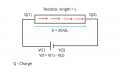# Why is electric field across a resistor constant, or voltage gradient linear ?

#### silv3r.m00n

Joined Apr 15, 2010
70
Hi

Consider this complex circuit :1. Consider an ideal resistor with uniform cross section and made of isotropic material with constant resistivity.
2. Consider an ideal battery giving a voltage difference of V across terminal.
3. Consider that the wires are superconductors with 0 resistance.

3. The voltage drop across the length of current flow is linear, that is del(V)/del(L) is constant.

4. This implies that Electrostatic force in the direction of current flow is constant throughout the resistor, since (vector)E = del(V)/del(L).

** Q: Is there a way to prove that the electric field is constant all along the length of the resistor in the direction of current flow ?

** Q: Or derive this mathematically using fundamental laws of electrostatics ?

** Q: Or prove why the del(V)/del(L) is linear across the length of the wire in the direction of the current flow ?

**Note:

1. Most other texts give the analogy of a capacitor with 2 charged plates, but i feel those examples are wrong, since they assume plates of infinite surface area which is clearly not analogous to the above circuit.

2. Don't tell E = V/L is constant at all points assuming V = IR (ohm's law), because its actually the other way. V=IR is probably derived from E = V/L being constant.

Last edited: